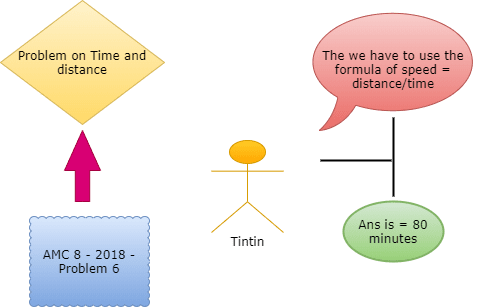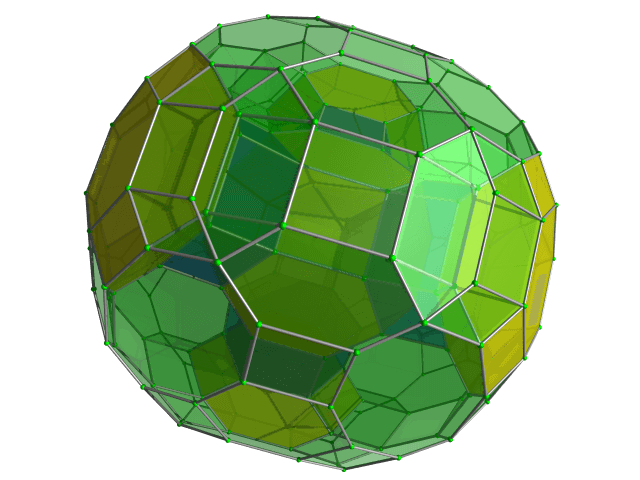Categories

# Time and Distance Problem from AMC 8: Problem 6 – 2018

Try this beautiful problem from AMC 8. It involves basic statistics and data representation. We provide sequential hints so that you can try the problem.

## Competency in Focus: Time and Distance calculation

This problem from American Mathematics Contest 8 (AMC 8, 2018) is based on calculation of time and distance. It is Question no. 6 of the AMC 8 2018 Problem series.

## First look at the knowledge graph:-## Next understand the problem

On a trip to the beach, Anh traveled 50 miles on the highway and 10 miles on a coastal access road. He drove three times as fast on the highway as on the coastal road. If Anh spent 30 minutes driving on the coastal road, how many minutes did his entire trip take? $\textbf{(A) }50\qquad\textbf{(B) }70\qquad\textbf{(C) }80\qquad\textbf{(D) }90\qquad \textbf{(E) }100$

### Basic Time and Distance problem with an easy interpretation from AMC 8 – 2018 – Problem 6

5/10
##### Suggested Book
Challenges and Thrills in Pre College Mathematics Excursion Of Mathematics

Do you really need a hint? Try it first!
Speed = $\frac {distance}{time}$  This can be the first hint for this sum. It is one of the important formula in science. Try to use it in this sum……..
So if we use the previous hint the speed would be  r = $\frac {d}{t}$ so , r = $\frac {10}{0.5}$    r = 20 mph.
His speed on the highway then is $60$ mph. He drives $50$ miles, so he drives for $\frac{5}{6}$ hours, which is equal to $50$ minutes. Note : 60 miles\hour is equal to 1 mile\minute
I think you already got the answer but if not here is the last hint. The total amount of minutes spent on his trip is  =80 minutes

## AMC - AIME Program

AMC - AIME - USAMO Boot Camp for brilliant students. Use our exclusive one-on-one plus group class system to prepare for Math Olympiad## External Tangent | AMC 10A, 2018 | Problem 15

Try this beautiful Problem on geometry based on circle from AMC 10A, 2018. Problem-15. You may use sequential hints to solve the problem.

## Dice Problem | AMC 10A, 2014| Problem No 17

Try this beautiful Problem on Probability from AMC 10A, 2014. Problem-17, You may use sequential hints to solve the problem.

## Problem on Curve | AMC 10A, 2018 | Problem 21

Try this beautiful Problem on Co-ordinate geometry from AMC 10A, 2018. Problem-21, You may use sequential hints to solve the problem.

## Right-angled Triangle | AMC 10A, 2018 | Problem No 16

Try this beautiful Problem on triangle from AMC 10A, 2018. Problem-16. You may use sequential hints to solve the problem.

## Finding Greatest Integer | AMC 10A, 2018 | Problem No 14

Try this beautiful Problem on Algebra from AMC 10A, 2018. Problem-14, You may use sequential hints to solve the problem.

## Length of the crease | AMC 10A, 2018 | Problem No 13

Try this beautiful Problem on triangle from AMC 10A, 2018. Problem-13. You may use sequential hints to solve the problem.

## Right-angled shaped field | AMC 10A, 2018 | Problem No 23

Try this beautiful Problem on triangle from AMC 10A, 2018. Problem-23. You may use sequential hints to solve the problem.

## Area of region | AMC 10B, 2016| Problem No 21

Try this beautiful Problem on Geometry on Circle from AMC 10B, 2016. Problem-20. You may use sequential hints to solve the problem.

## Coin Toss Problem | AMC 10A, 2017| Problem No 18

Try this beautiful Problem on Probability from AMC 10A, 2017. Problem-18, You may use sequential hints to solve the problem.

## GCF & Rectangle | AMC 10A, 2016| Problem No 19

Try this beautiful Problem on Geometry on Rectangle from AMC 10A, 2010. Problem-19. You may use sequential hints to solve the problem.

This site uses Akismet to reduce spam. Learn how your comment data is processed.